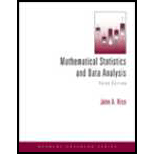# Show that the set function Q ( A ) = P ( A | B ) satisfies the axioms for a probability measure if B is an event with P ( B ) &gt; 0 . Show that P ( A ∪ C | B ) = P ( A | B ) + P ( C | B ) − P ( A ∩ C | B ) .### Mathematical Statistics and Data A...

3rd Edition
John A. Rice
Publisher: Cengage Learning
ISBN: 9781111793715### Mathematical Statistics and Data A...

3rd Edition
John A. Rice
Publisher: Cengage Learning
ISBN: 9781111793715

#### Solutions

Chapter 1.8, Problem 64P
To determine

## Expert Solution

### Want to see the full answer?

Check out a sample textbook solution.

### Want to see this answer and more?

Experts are waiting 24/7 to provide step-by-step solutions in as fast as 30 minutes!*

*Response times may vary by subject and question complexity. Median response time is 34 minutes for paid subscribers and may be longer for promotional offers.